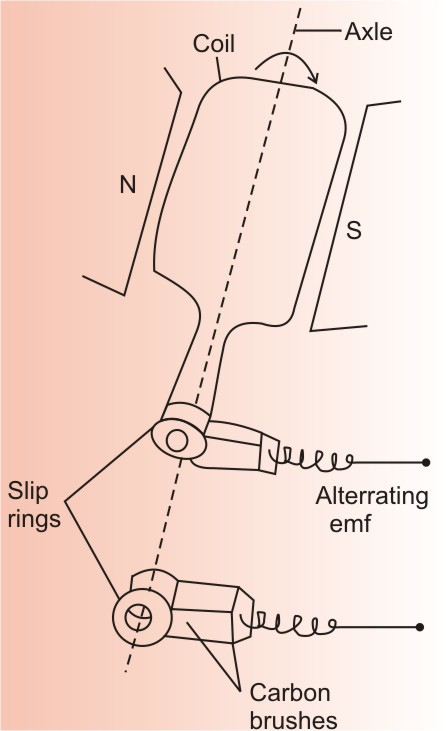# (a)  Draw a schematic diagram of an ac generator. Explain its working and obtain the expression for the instantaneous value of the emf in terms of the magnetic field B,number of turns N of the coil of area A rotating with angular frequency . Show how an alternating emf is generated by a loop of write rotating in a magnetic field.  (b) A circular coil of radius 10 cm and 20 turns is rotated about its vertical diameter with angular speed of 50 rad  in a uniform horizontal magnetic field of  (i) calculate the maximum and average emf induced in the coil. (ii) If the coil forms a closed loop of resistance 10, calculate the maximum current in the coil and the average power loss due to Joule heating.

working of Ac generator:-
Systematic Diagram of Ac generator:whenever a coil is placed in a uniform magnetic field and rotated, the flux linked with the coil changes and an emf induces in the coil
The ends of the coil are connected to an external circuit by means of slip rings and brushes. when, the flux linked with the coil of area(A), placed in a uniform magnetic field (B)'
then, the flux

or
the emf induced in the coil is given as :-
where, N= no. of turns.

(b) (i) we have given the values:

we know, the induced emf

The average value of induced emf=0
(ii) To calculate the maximum current
we have
is absolute permittivity, R is resistance.

To determine the average power loss, we have

### Preparation Products

##### Knockout NEET July 2020

An exhaustive E-learning program for the complete preparation of NEET..

₹ 15999/- ₹ 6999/-
##### Rank Booster NEET 2020

This course will help student to be better prepared and study in the right direction for NEET..

₹ 9999/- ₹ 4999/-
##### Knockout JEE Main July 2020

An exhaustive E-learning program for the complete preparation of JEE Main..

₹ 12999/- ₹ 6999/-
##### Test Series NEET July 2020

Take chapter-wise, subject-wise and Complete syllabus mock tests and get in depth analysis of your test..

₹ 4999/- ₹ 2999/-